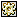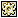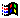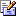Contents Articles Behaviors Books Director News Director Web Sites FAQ Games Mailing Lists News Groups Project Examples Reviews Software Tools Useful Web Sites Utilities Xtras

 Don't miss these#Bouncing Ball

 Compatibilities:This item has not yet been rated

Author: MikeFagan

A behaviour to animate a bouncing ball using x:y co-ordinates rather than trigonometry Some of the code courtesy Direct-L. last Modification = 8:10 PM 17/10/97 MAF

 property mySprite     -- the sprite Number of current sprite property mySelf       -- the sprite me property myWidth      -- the width of the animated sprite property myHeight     -- the height of the animated sprite property velocityV    -- the vertical velocity of the sprite property velocityH    -- the horizontal velocity of the sprite property speed        -- to set variations of speed of the sprite property LastTicks    -- the tick count last time on enterframe property randomSprite -- set sprite to random point at start if set property collisionOn  -- set collision detection property direction    -- direction sprite travelling in when hit property hitMe        -- which side did I get hit from property stOffset     -- offset for edge of stage -- initialise Properties on beginSprite me      -- get the number, the width and the height of my sprite   set mySprite = the SpriteNum of me   set mySelf = sprite (the spriteNum of me)   set myWidth  = the width of sprite mySprite   set myHeight = the height of sprite mySprite      -- set the speed of the objects   set velocityV = random(speed)   set velocityH = random(speed)   set LastTicks = 0      -- assign a random starting location for the object   if randomSprite = true then     set stageHeight = the stageBottom - the stageTop     set stageWidth = the stageRight - the stageLeft     set the locV of sprite mySprite to random((stageHeight) - 100) + 50     set the locH of sprite mySprite to random((stageWidth) - 100) + 50   end if      -- add the sprite number to global list   addAt the actorList mySprite, mySprite    end beginSprite -- End processes on endSprite me      -- clean up the spritelist   deleteOne the actorList mySprite    end endSprite -- Animate the Sprite on enterFrame me      --save the ticks   set LastTicks = (the ticks)      -- increment position of sprite based on its velocity   set currentV = the locV of sprite mySprite + velocityV   set currentH = the locH of sprite mySprite + velocityH      -- check to see if it is off the top or bottom of the stage   -- turn it around if it is      if currentV < (integer(myHeight/2) + stOffset) then     set velocityV = -1 * velocityV     set currentV = (integer(myHeight/2) + stOffset)   end if      if currentV > (the stageBottom - the stageTop) - (integer(myHeight/2) + stOffset) then     set velocityV = -1 * velocityV     set currentV = (the stageBottom - the stageTop) - (integer(myHeight/2) + stOffset)   end if      -- check to see if it is off the left or right of the stage   -- turn it around if it is   if currentH < (integer(myWidth/2) + stOffset) then     set velocityH = -(1 * velocityH)     set currentH = (integer(myWidth/2) + stOffset)   end if   if currentH > (the stageRight - the stageLeft) - (integer(myWidth/2) + stOffset) then     set velocityH = -(1 * velocityH)     set currentH = (the stageRight - the stageLeft) - (integer(myWidth/2) + stOffset)   end if      -- update its location on the stage   set the loc of sprite mySprite = point(currentH,currentV)      -- if collision detection on   if collisionOn = true then          -- collision detection procedure between sprites     collisionDetect   end if    end enterFrame -- detect collision of two sprites on collisionDetect      -- check all sprites with this behaviour   repeat with listPos = 1 to count(the actorList)     set otherSprite = getOne(the actorList, listPos)          -- if the other sprite isn't referring to me     if otherSprite <> mySprite then              -- check to see it is within my circle       -- uses a circle with radius of half sprite width       circleIntersect mySprite otherSprite       if the result = TRUE then         -- check for how collision occurred           checkCollision otherSprite       end if     end if   end repeat    end collisionDetect -- check whether sprite is within a specified circle of another sprite on circleIntersect sprite1, sprite2      -- sprite1 and sprite2 are channel numbers containing circle sprites   -- first get the radii of the circles   set circle1Radius = the width of sprite sprite1 / 2   set circle2Radius = the width of sprite sprite2 / 2      -- next determine the required distance between the two centerpoints for non-intersection   set minDistanceForNonIntersect = circle1Radius + circle2Radius      -- now determine the actual distance between the centerpoints of the two sprites   set actualDistance = distance (the loc of sprite sprite1 + point(circle1Radius, circle1Radius), the loc of sprite sprite2 + point(circle2Radius, circle2Radius))      -- now compare the two and return if within circle   if actualDistance > minDistanceForNonIntersect then     return FALSE   else     return TRUE   end if    end circleIntersect -- compute distance between two points on distance point1, point2   set deltaH = the locH of point1 - the locH of point2   set deltaV = the locV of point1 - the locV of point2   --return actual distance between the two points   return sqrt(deltaH * deltaH + deltaV * deltaV) end distance -- check rects of sprites and overlap for collision direction on checkCollision otherSprite      -- the sprite can be travelling in 1 of 8 directions & can be hit from 1 of 8 directions.   -- calculate 8 segments of circle as 1 through 8 from velocityH & velocityV   -- calculate the 8 directions sprite can be hit from by subtracting Loc of other sprite   -- from my sprite and using locH and locV values of the result.      -- calculate the direction in which the sprite is hit from by another sprite   set delta = the loc of sprite mySprite - the loc of sprite otherSprite   set deltaX = the locH of delta        -- horizontal difference between sprites   set deltaY = the locV of delta        -- vertical difference between sprites      if deltaX >= 0 then     if deltaY >= 0 then       if abs(deltaX) >= abs(DeltaY) then set hitMe = 1       else set hitMe = 2     else       if abs(deltaX) >= abs(DeltaY) then set hitMe = 8       else set hitMe = 7     end if   else     if deltaY >= 0 then       if abs(deltaX) >= abs(DeltaY) then set hitMe = 4       else set hitMe = 3     else       if abs(deltaX) >= abs(DeltaY) then set hitMe = 5       else set hitMe = 6     end if   end if      -- calculate the direction in which the sprite is travelling   if velocityH <= 0 then     if velocityV <= 0 then       if abs(velocityH) >= abs(velocityV) then set direction = 1       else set direction = 2     else       if abs(velocityH) >= abs(velocityV) then set direction = 8       else set direction = 7     end if   else     if VelocityV <= 0 then       if abs(velocityH) >= abs(velocityV) then set direction = 4       else set direction = 3     else       if abs(velocityH) >= abs(velocityV) then set direction = 5       else set direction = 6     end if   end if      -- set x and y to absolute values of velocityH and velocityV for ease of calculation   set x = abs(velocityH)   if x = 0 then set x = 1   -- set to 1 to avoid divide by 0 errors   set y = abs(velocityV)   if y = 0 then set y = 1   -- set to 1 to avoid divide by 0 errors      case direction of     1:           case hitMe of         1:           set velocityH = x           set velocityV = x - y         2:           set velocityH = -1 * (x * y/x)           set velocityV = y * x/y         4,3:           set velocityV = y         5:           set velocityV = -1 * (x - y)         6,7:           set velocityH = -1 * (x * y/x)           set velocityV = -1 * (y * x/y)         8:           set velocityH = x * y/x           set velocityV = -1 * (y * x/y)         end case         2:         case hitMe of         2:           set velocityH = y - x           set velocityV = y         3:           set velocityH = -1 * (x * y/x)           set velocityV = y * x/y           5,4:           set velocityH = -1 * (x * y/x)           set velocityV = -1 * (y * x/y)         6:           set velocityH = -1 * (y - x)         7,8:           set velocityH = x           1:           set velocityH = x * y/x           set velocityV = -1 * (y * x/y)       end case     3:           case hitMe of         2:           set velocityH = x * y/x           set velocityV = y * x/y           3:           set velocityH = -1 * (y - x)           set velocityV = y         4:           set velocityH = -1 * (x * y/x)           set velocityV = -1 * (y * x/y)         6,5:           set velocityH = -1 * x         7:           set velocityH = y - x           8,1:           set velocityH = x * y/x           set velocityV = -1 * (y * x/y )       end case     4:           case hitMe of         1,2:           set velocityV = y         3:           set velocityH = x * y/x           set velocityV = y * x/y           4:           set velocityH = -1 * x           set velocityV = x - y         5:           set velocityH = -1 * (x * y/x)           set velocityV = -1 * (y * x/y)         7,6:           set velocityH = x * y/x           set velocityV = -1 * (y * x/y)           8:           set velocityV = -1 * (x - y)       end case     5:           case hitMe of         1:           set velocityV = x - y         2,3:           set velocityH = x * y/x           set velocityV = y * x/y           4:           set velocityH = -1 * (x * y/x)           set velocityV = y * x/y         5:           set velocityH = -1 * x           set velocityV = -1 * (x - y)         6:           set velocityH = x * y/x           set velocityV = -1 * (y * x/y)           8,7:           set velocityV = -1 * y       end case       6:           case hitMe of         1,8:           set velocityH = x * y/x           set velocityV = y * x/y         2:           set velocityH = y - x           3,4:           set velocityH = -1 * x         5:           set velocityH = -1 * (x * y/x)           set velocityV = y * x/y           6:           set velocityH = -1 * (y - x)             set velocityV = -1 * y         7:           set velocityH = x * y/x           set velocityV = -1 * (y * x/y)       end case     7:           case hitMe of         8:           set velocityH = x * y/x           set velocityV = y * x/y         2,1:           set velocityH = x         3:           set velocityH = -1 * (y - x)         4,5:           set velocityH = -1 * (x * y/x)           set velocityV = y * x/y           6:           set velocityH = -1 * (x * y/x)           set velocityV = -1 * (y * x/y)         7:           set velocityH = y - x           set velocityV = -1 * y       end case     8:           case hitMe of         1:           set velocityH = x * y/x           set velocityV = y * x/y           3,2:           set velocityH =  -1 * (x * y/x)           set velocityV =  y * x/y         4:           set velocityV = x - y         5,6:           set velocityV = -1 * y         7:           set velocityH = -1 * (x * y/x)           set velocityV = -1 * (y * x/y)         8:           set velocityH = x           set velocityV = -1 * (x - y)       end case   end case   end checkCollision -- create a Property List on getPropertyDescriptionList     set p_list = [#speed: [ #default:10, #format:#integer, #comment:"Set the sprite speed",#range: [#min:0,#Max:30]],¬ #stOffset: [ #default:0, #format:#integer, #comment:"Offset for the edge of stage",#range: [#min:0,#Max:50]],¬ #randomSprite:[#default:1,#format:#boolean,#comment:"Start from random point?"],¬ #collisionOn:[#default:1,#format:#boolean,#comment:"Collision detection on?"]]   return p_list   end getPropertyDescriptionList -- create a Behaviour Description on getBehaviorDescription     put "A sprite behaviour that bounces the sprite around the screen like a frictionless " into line1   put "ball. Collision detection can be switched on that allows sprites to bounce of " into line2   put "one another. Animation speed is set by the number of pixels. " &RETURN & RETURN  into line3   put "There are also other functions to modify this behaviour: " & RETURN into line4   put "1. Stage Offset - to inset the edge of the screen used. " & RETURN into line5   put "2. Random start - sprite starts from random point. " into line6   return line1 & line2 & line3 & line4 & line5 & line6   end getBehaviorDescription

 Contact MMI 36 South Court Sq Suite 300 Newnan, GA 30263 USASend e-mail